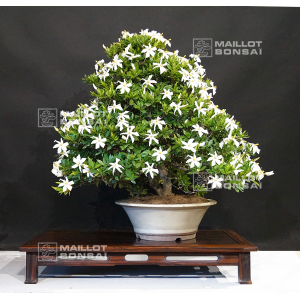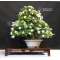##### The Japanese Bonsai specialist
Direct order Contact Help / Services Newsletter# Gardenia jasminoides bonsai ref:12070171

Gardenia jasminoidesref. : 10209Product unavailable

###### Description

Height of the tree only: 61 cm.

Length of the bough: 70 cm.
Quality pot Ø 36*13 cm.

Sold without presentation shelf.

What could be more divine than catching the scent of this white flowering gardenia jasminoides bonsai? It's from the rubiaceae family of gardenia and is called kushinashi in Japanese. The shrub has continuously gleaming green foliage and the flower colour varies from cream to ivory to slightly pink, sending off a pungent aroma around the garden. It blooms steadily from the middle of June through to the end of July. Potted gardenias like to be kept in cold greenhouses or orangeries, but most of all it's important to protect them from frost during winter.

#gardenia 4.8 #bonsai 4.6 #from 4.4 #jasminoides 4.1 #masterpieces 3.2 #presentation 2.2 #continuously 2.2 #greenhouses 2.1 #kushinashi 2 #orangeries 2

Formule
(( ROUND((CHAR_LENGTH(b.article_nom)-CHAR_LENGTH(REPLACE(b.article_nom, 'gardenia', '')))/LENGTH('gardenia')) + ROUND((CHAR_LENGTH(b.article_description)-CHAR_LENGTH(REPLACE(b.article_description, 'gardenia', '')))/LENGTH('gardenia')) ) * 4.8) + (( ROUND((CHAR_LENGTH(b.article_nom)-CHAR_LENGTH(REPLACE(b.article_nom, 'from', '')))/LENGTH('from')) + ROUND((CHAR_LENGTH(b.article_description)-CHAR_LENGTH(REPLACE(b.article_description, 'from', '')))/LENGTH('from')) ) * 4.4) + (( ROUND((CHAR_LENGTH(b.article_nom)-CHAR_LENGTH(REPLACE(b.article_nom, 'jasminoides', '')))/LENGTH('jasminoides')) + ROUND((CHAR_LENGTH(b.article_description)-CHAR_LENGTH(REPLACE(b.article_description, 'jasminoides', '')))/LENGTH('jasminoides')) ) * 4.1) + (( ROUND((CHAR_LENGTH(b.article_nom)-CHAR_LENGTH(REPLACE(b.article_nom, 'bonsai', '')))/LENGTH('bonsai')) + ROUND((CHAR_LENGTH(b.article_description)-CHAR_LENGTH(REPLACE(b.article_description, 'bonsai', '')))/LENGTH('bonsai')) ) * 2.6) + (( ROUND((CHAR_LENGTH(b.article_nom)-CHAR_LENGTH(REPLACE(b.article_nom, 'presentation', '')))/LENGTH('presentation')) + ROUND((CHAR_LENGTH(b.article_description)-CHAR_LENGTH(REPLACE(b.article_description, 'presentation', '')))/LENGTH('presentation')) ) * 2.2) + (( ROUND((CHAR_LENGTH(b.article_nom)-CHAR_LENGTH(REPLACE(b.article_nom, 'continuously', '')))/LENGTH('continuously')) + ROUND((CHAR_LENGTH(b.article_description)-CHAR_LENGTH(REPLACE(b.article_description, 'continuously', '')))/LENGTH('continuously')) ) * 2.2) + (( ROUND((CHAR_LENGTH(b.article_nom)-CHAR_LENGTH(REPLACE(b.article_nom, 'greenhouses', '')))/LENGTH('greenhouses')) + ROUND((CHAR_LENGTH(b.article_description)-CHAR_LENGTH(REPLACE(b.article_description, 'greenhouses', '')))/LENGTH('greenhouses')) ) * 2.1) + (( ROUND((CHAR_LENGTH(b.article_nom)-CHAR_LENGTH(REPLACE(b.article_nom, 'orangeries', '')))/LENGTH('orangeries')) + ROUND((CHAR_LENGTH(b.article_description)-CHAR_LENGTH(REPLACE(b.article_description, 'orangeries', '')))/LENGTH('orangeries')) ) * 2) + (( ROUND((CHAR_LENGTH(b.article_nom)-CHAR_LENGTH(REPLACE(b.article_nom, 'kushinashi', '')))/LENGTH('kushinashi')) + ROUND((CHAR_LENGTH(b.article_description)-CHAR_LENGTH(REPLACE(b.article_description, 'kushinashi', '')))/LENGTH('kushinashi')) ) * 2) + (( ROUND((CHAR_LENGTH(b.article_nom)-CHAR_LENGTH(REPLACE(b.article_nom, 'flowering', '')))/LENGTH('flowering')) + ROUND((CHAR_LENGTH(b.article_description)-CHAR_LENGTH(REPLACE(b.article_description, 'flowering', '')))/LENGTH('flowering')) ) * 1.9)

## Secure payment## Delivery

Our logistic partners :